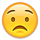# Credit Management Practise Exam Task 4

Hi,

I'm hoping someone can help me as I'm really stuck on how to complete this question, I'm unsure how to work out the difference in the % between the years I've looked through my books and online but i cannot seem to come back to these answers.

Really hoping someone can help.

Thanks
Sophie

• The gross profit has fallen in absolute terms by:
(14,177 / 17,215) - 1 = -0.17647 = 17.65% fall

and the increase of:

[(8,598 + 5,595) / (7,535 + 5,357)] - 1 =
(14,193 / 12,892) - 1 = 0.1009 = 10.09%

in combined distribution and administration costs
• hal978 said:

The gross profit has fallen in absolute terms by:
(14,177 / 17,215) - 1 = -0.17647 = 17.65% fall

and the increase of:

[(8,598 + 5,595) / (7,535 + 5,357)] - 1 =
(14,193 / 12,892) - 1 = 0.1009 = 10.09%

in combined distribution and administration costs

thanks so much for replying without sounding silly where do you get the -1 from?
• The result would be a ratio or an index if I don't take out the 1 (e.g. 0.8235 or 1.1009). So to convert it to a percentage change, 1 has to be deducted.

Alternatively it could be calculated like this:

[(14,177 - 17,215) / 17,215] = -3,308 / 17,215 = -0.17647 = 17.65% fall
• hal978 said:

The result would be a ratio or an index if I don't take out the 1 (e.g. 0.8235 or 1.1009). So to convert it to a percentage change, 1 has to be deducted.

Alternatively it could be calculated like this:

[(14,177 - 17,215) / 17,215] = -3,308 / 17,215 = -0.17647 = 17.65% fall

thank you for helping me with this, the whole task is a bit if a struggle for medo you have any tips for this task?
• would anyone be able to tell me how they got to this gearing ratio answer please?
• would anyone be able to tell me how they got to this gearing ratio answer of 233.80 please
• would anyone be able to tell me how they got to this gearing ratio answer please?

would anyone be able to tell me how they got to this gearing ratio answer of 233.80 please

does anybody have any idea please?
• Hi Sophie

I didn't do the credit management module. But from the financial statements and D&C.

The Gearing ratio is - Debt/Equity *100

(21500+2750)/10372 *100 = 233.80

I hope this helps might be worth checking the Credit management follows the same rules.
AAT Level 4, MAAT
ACCA in progress
F4- Passed Aug 2020
F5- Passed Dec 2020
F6- Passed Sep 2020
F7- Passed June 2021
F8 - Passed Sep 2021
F9 - Passed June 2021

SBL
SBR - Passed Mar 22
ATX - Passed Dec 21
APM -
• Pian32 said:

Hi Sophie

I didn't do the credit management module. But from the financial statements and D&C.

The Gearing ratio is - Debt/Equity *100

(21500+2750)/10372 *100 = 233.80

I hope this helps might be worth checking the Credit management follows the same rules.

I assume that they will also accept D/D+E *100 also? As this calculation gives a completely different figure obviously.
• Hi, sorry to jump in but I got stuck on the same part too. Does anyone know if D/D+E would be accepted as the question does not state different? Also I am struggling to find the credit terms (attached), any help would be appreciated.

Thanks
• Hi, sorry to jump in but I got stuck on the same part too. Does anyone know if D/D+E would be accepted as the question does not state different? Also I am struggling to find the credit terms (attached), any help would be appreciated.

Thanks
• Hi, sorry to jump in but I got stuck on the same part too. Does anyone know if D/D+E would be accepted as the question does not state different? Also I am struggling to find the standard payment terms at the end of the liquidity part, any help would be appreciated.

Thanks
• It can be:

Total debt ÷ (total debt + equity) x 100

Or long term debt could be used instead of total debt. The exam usually states what formula to use to calculate gearing.

If total debt is used, remember it only includes the borrowings/loans in the current and non-current liabilities. Don't include any trade payables.

Good luck.